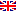Scheda programma d'esame
ANALISI NUMERICA CON LABORATORIO
DARIO ANDREA BINI
CdSMATEMATICA
Codice043AA
CFU9
PeriodoPrimo semestre
LinguaItaliano

ModuliSettore/iTipoOreDocente/i
ANALISI NUMERICAMAT/08LEZIONI81
 LIDIA ACETO unimap DARIO ANDREA BINI unimap BEATRICE MEINI unimapEsporta in pdf
Learning outcomes
Knowledge

The goal of the course is twofold. From one hand, it aims to stimulate the interest about the algorithmic point of view of mathematical problems. On the other hand, it aims to create a sound basis of theoretical tools and numerical methods which enable the student to solve basic problems in an algorithmic way and to create the background for learning more advanced computational tools. The student who successfully completes the course will be also able to implement numerical algorithms in the language Octave / Matlab

Assessment criteria of knowledge

The student will be assessed on his/her demonstrated ability

•  to discuss the main course contents using the appropriate terminology,
• to report properties, theorems and their proofs - to solve exercises
• to implement algorithms in the language Octave / Matlab

Methods:

• Final oral exam
• Final written exam
Skills

At the end of the course students will have capability of anaiyzing numerical problems from the computational point of view and to provide Octave / Matlab implementation of numerical algorithms.

Assessment criteria of skills

The assessment criteria of skills rely on  the implementation of  Matlab / Octave functions concerning specific problems, and on solving suitable exercises concerning different parts of the course.

Behaviors

The students will reach a numerical thought where the concepts of numerical stability and conditioning play a fundamental role, and where minimizing the computational complexity  is a continuous demand in the design and analysis of numerical algorithms.

Assessment criteria of behaviors

The implementation of  Matlab / Octave functions concerning specific problems, and solving suitable exercises concerning different  parts of the course are once again the main criteria for the assessment of behaviors.

Prerequisites

Basic notions of Linear Algebra and of Calculus.

Teaching methods

Frontal lectures concern theory and exercises. They are performed in a classroom with chalk and blackboard.

Laboratory is performed in a classroom with computers and beamer.

The course has its own e-learning site where the student can download lecture notes, exercises, find the texts and the solutions of the past written exams, or, if requested, can upload exercises.

Attendance at the lectures is advised. Attendance at the laboratory is mandatory. If the student does not attend at least 9 out of 11 laboratory lessons then in the oral exam he/she is required to answer additional questions concerning the laboratory part.

Syllabus

- Rounding error analysis: numerical stability and conditioning - Numerical Linear Algebra: the Schur form, Gerschgorin theorems, norms - Direct methods for solving linear systems - Iterative methods for solving linear systems - Solving nonlinear equations: fixed point iterations - Interpolation and numerical integration - FFT and its applications - The language Octave

Bibliography

Recommended reading includes the following works:

• D.A. Bini, M. Capovani, O. Menchi, "Metodi numerici per l'algebra lineare", Zanichelli, 1988.
• R. Bevilacqua, D.A. Bini, M. Capovani, O. Menchi, Metodi Numerici, Zanichelli, 1992

• Additional lectures notes are supplied by the lecturers.

Assessment methods

The exam consists of a written and of an oral part

The written part generally consists of three exercises, one in the area of numerical linear algebra, one in the area of nonlinear problems, while the third one concerns the algorithmic analysis of a specific problem with the implementation of a solution algorithm in the syntax of Octave / Matlab. The exam is passed if the student solves at least 2 out of 3 exercises in an acceptable form. If the written exam is passed then the student has access to the oral exam.

The oral part generally consists of two questions on different parts of the program. The exam is passed if the student provides acceptable answers to both questions.

If the student has not solved the implementation exercise in the written part, then in the oral exam he/she will be requested to solve an additional implementation exercise.

Pagina web del corso

https://elearning.dm.unipi.it

Ultimo aggiornamento 27/07/2017 15:26